# Physics 2 April 27 2017 P 3 Challenge

• Slides: 10Physics 2 – April 27, 2017 P 3 Challenge – A rotating fan completes 1200 revolutions every minute. Consider the tip of a blade, at a radius of 0. 15 m. a) Through what distance does the tip move in one revolution? Wat are b) the tip’s speed and c) the magnitude of its acceleration? d) what is the period of the motion?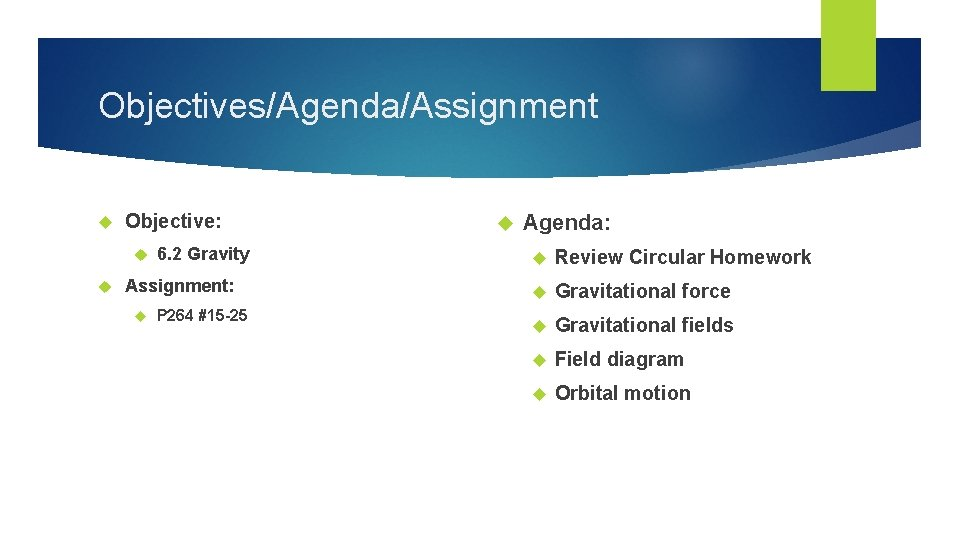Objectives/Agenda/Assignment Objective: 6. 2 Gravity Assignment: P 264 #15 -25 Agenda: Review Circular Homework Gravitational force Gravitational fields Field diagram Orbital motionGravitational Force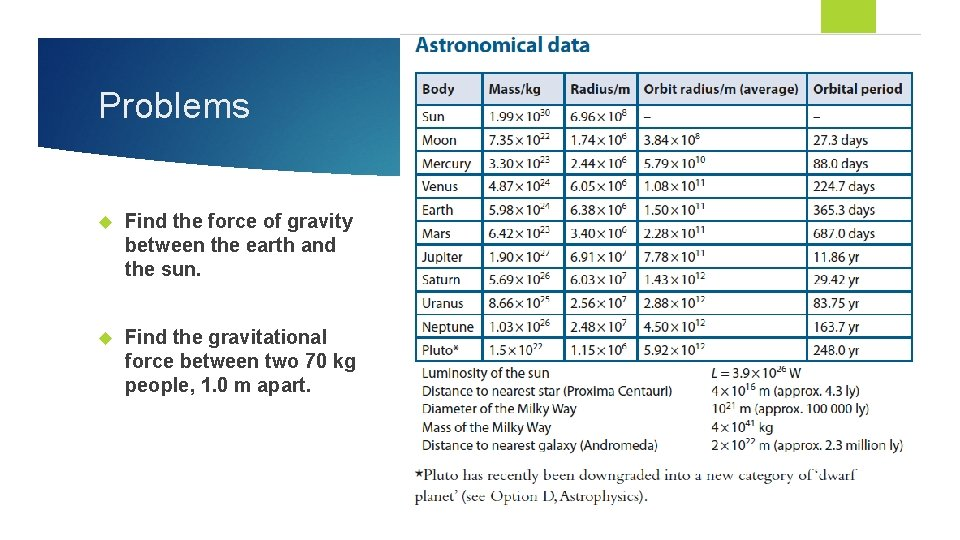Problems Find the force of gravity between the earth and the sun. Find the gravitational force between two 70 kg people, 1. 0 m apart.Acceleration due to gravityGravity fields Gravitational forces apply over a distance. When a force has an effect without direct contact, it is a field force. Gravitational fields are represented by radial lines that point toward the center of mass that is creating a gravitational acceleration. The lines are evenly distributed, indicating that the force is uniform in all directions. The density of lines represents the magnitude of the force. It is highest close to the mass, and decreases farther away.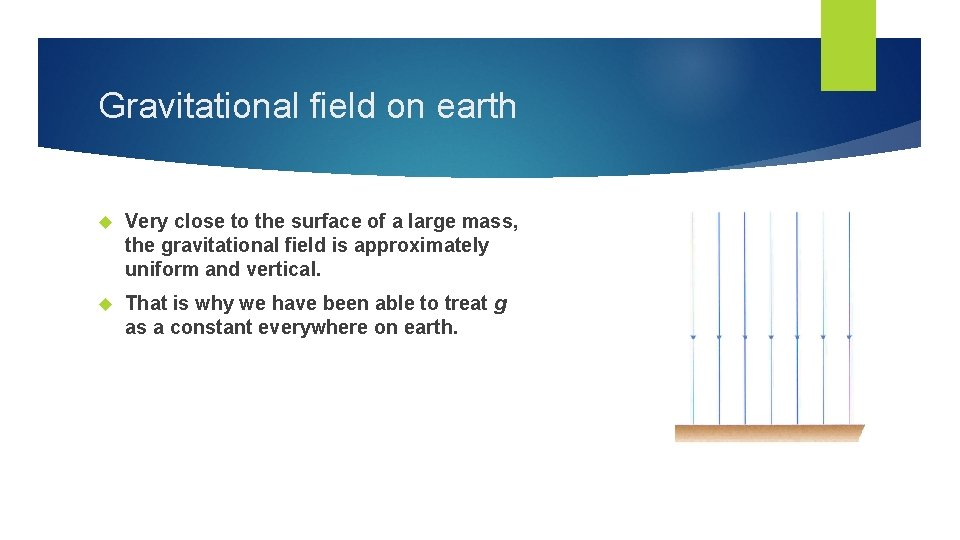Gravitational field on earth Very close to the surface of a large mass, the gravitational field is approximately uniform and vertical. That is why we have been able to treat g as a constant everywhere on earth.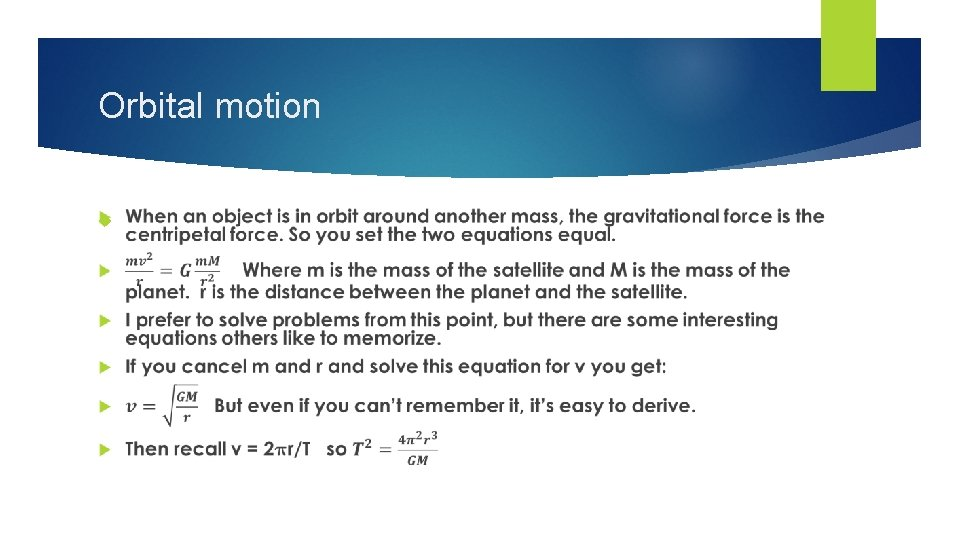Orbital motion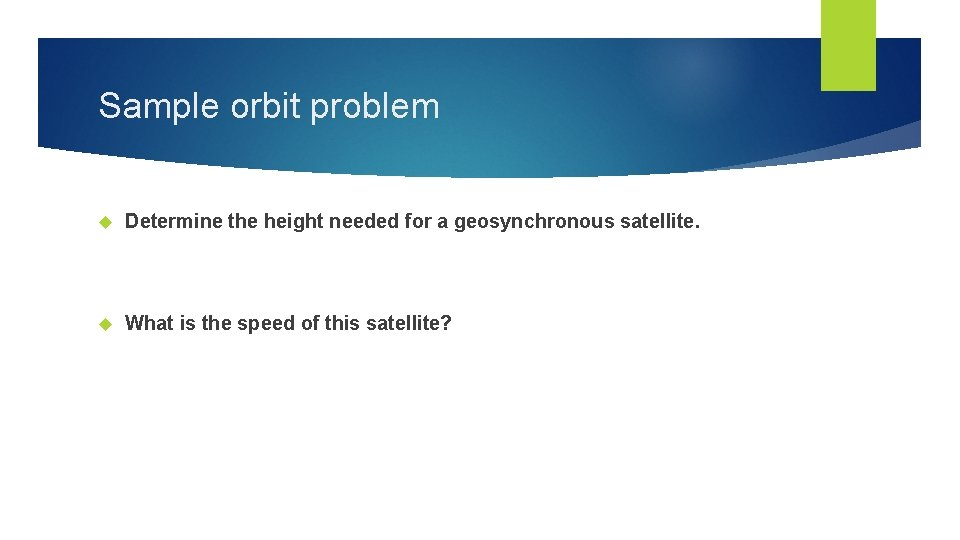Sample orbit problem Determine the height needed for a geosynchronous satellite. What is the speed of this satellite?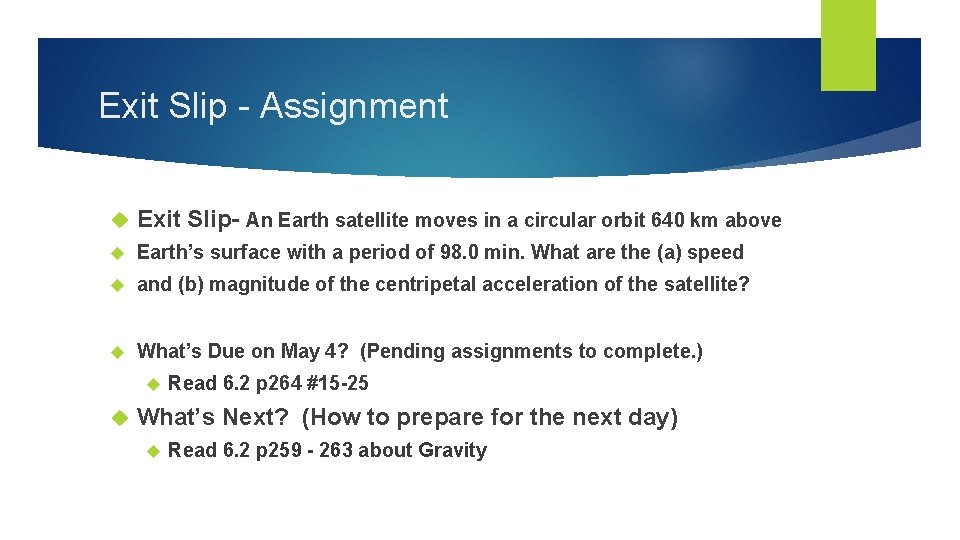Exit Slip - Assignment Exit Slip- An Earth satellite moves in a circular orbit 640 km above Earth’s surface with a period of 98. 0 min. What are the (a) speed and (b) magnitude of the centripetal acceleration of the satellite? What’s Due on May 4? (Pending assignments to complete. ) Read 6. 2 p 264 #15 -25 What’s Next? (How to prepare for the next day) Read 6. 2 p 259 - 263 about Gravity